This Mathcad worksheet takes two number sequences, xn and hn and performs their convolution.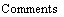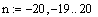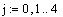n is the range over which we will define the number sequences.
Note that the worksheet ORIGIN value has to be set to encompass
the -20 so that Mathcad understands the negative subscripts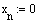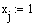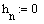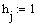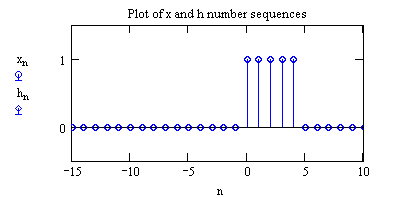j is the range of sample points where the functions are 1
The two sequences are the same for this example. They
absolutely don't have to be.
Step 1 is to flip one of the sequences, hn, about the y axis and then shift it to the left an
arbitrary N0 of samples.
N0 is arbirtrary number of samples hn shifted to left before convolution. Make sure ORIGIN set large enough negative to support.

hS is the flipped and shifted sequence.

The N0 shift will be removed in the step below. It is used here to show that the number sequence should be moved far enough to the left to ensure no overlap exists between the two sequences as the convolution begins.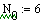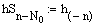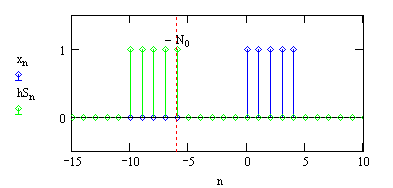Shift the "sliding" number sequence so that its right edge overlaps at 1 sample point
with the other number sequence.
Shift the hn sequence so its right edge overlaps at one sample point with the left edge of the xn sequence. This is not necessary for a proper convolution result, it is only necessary for this worksheet so that the convolution result begins at the first sample point of the x sequence. If the hSS sequence is set equal to the hS sequence the convolution, while still correctly calculated, the results will begin to occur N0 samples to the right.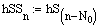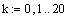Perform convolution calculation.
The upper limit of k just needs to be far enough to the right to ensure no more overlap occurs between the two sequences. the hSS sequence has been completed slid across the x.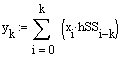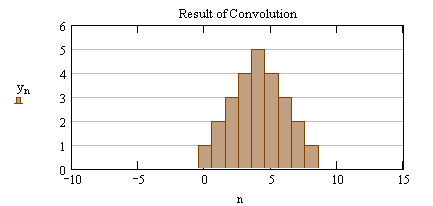yk is a summation of the products up to k at each sample point k. The result of the convolution at that sample point depends on the product of the two sequences at that sample point plus all those results prior. The i-k subscript on the hS sequence has the effect of "sliding" the hS sequence across the x sequence as products are computed.
Russell W. Patterson, P.E.
Manager, System Protection & Analysis
Tennessee Valley Authority
rwpatterson@tva.gov